Next: Q-learning in Generalized MDPs Up: Preliminaries Previous: Markov Decision Processes with

## Generalized Markov Decision Processes

[Szepesvári and Littman(1996)] have introduced a more general model. Their basic concept is that in the Bellman equations, the operation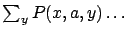(i.e., taking expected value w.r.t. the transition probabilities) describes the effect of the environment, while the operation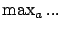describes the effect of an optimal agent (i.e., selecting an action with maximum expected value). Changing these operators, other well-known models can be recovered.

A generalized MDP is defined by the tuple, where X, A, R are defined as above;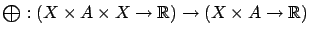is an expected value-type" operator and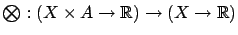is a maximization-type" operator. For example, by settingand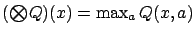(where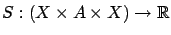and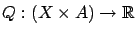), the expected-reward MDP model appears.

The task is to find a value function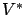satisfying the abstract Bellman equations:or in short form: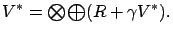The optimal value function can be interpreted as the total reward received by an agent behaving optimally in a non-deterministic environment. The operator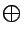describes the effect of the environment, i.e., how the value of taking action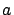in statedepends on the (non-deterministic) successor state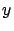. The operator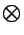describes the action-selection of an optimal agent. When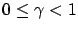, and bothandare non-expansions, the optimal solutionof the abstract Bellman equations exists and it is unique.

The great advantage of the generalized MDP model is that a wide range of models, e.g., Markov games [Littman(1994)], alternating Markov games [Boyan(1992)], discounted expected-reward MDPs [Watkins and Dayan(1992)], risk-sensitive MDPs [Heger(1994)], exploration-sensitive MDPs [John(1994)] can be discussed in this unified framework. For details, see the work of [Szepesvári and Littman(1996)].

SubsectionsNext: Q-learning in Generalized MDPs Up: Preliminaries Previous: Markov Decision Processes with